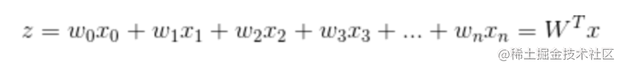# 机器学习之逻辑回归(纯python实现)

logistic回归是一种广义的线性回归，通过构造回归函数，利用机器学习来实现分类或者预测。

### 原理

1. 预测函数(h)。该函数就是分类函数，用来预测输入数据的判断结果。过程非常关键，需要预测函数的“大概形式”， 比如是线性还是非线性的。 本文参考机器学习实战的相应部分，看一下数据集。
``````// 两个特征
-0.017612   14.053064   0
-1.395634   4.662541    1
-0.752157   6.538620 0
-1.322371   7.152853    0
0.423363 11.054677   0
0.406704    7.067335    1

1. Cost函数(损失函数)：该函数预测的输出h和训练数据类别y之间的偏差，(h-y)或者其他形式。综合考虑所有训练数据的cost， 将其求和或者求平均，极为J函数， 表示所有训练数据预测值和实际值的偏差。

2. 显然，J函数的值越小，表示预测的函数越准确(即h函数越准确)，因此需要找到J函数的最小值。有时需要用到梯度下降。

### 具体过程

#### 构造预测函数向量x是特征变量, 是输入数据。此数据有两个特征，可以表示为z = w0x0 + w1x1 + w2x2。w0是常数项，需要构造x0等于1(见后面代码)。 向量W是回归系数特征，T表示为列向量。 之后就是确定最佳回归系数w(w0, w1, w2)。

#### cost函数

``````# sigmoid函数和初始化数据
def sigmoid(z):
return 1 / (1 + np.exp(-z))

def init_data():
dataMatIn = data[:, 0:-1]
classLabels = data[:, -1]
dataMatIn = np.insert(dataMatIn, 0, 1, axis=1)  #特征数据集，添加1是构造常数项x0
return dataMatIn, classLabels

``````//  梯度上升
dataMatrix = np.mat(dataMatIn)  #(m,n)
labelMat = np.mat(classLabels).transpose()
m, n = np.shape(dataMatrix)
weights = np.ones((n, 1))  #初始化回归系数（n, 1)
alpha = 0.001 #步长
maxCycle = 500  #最大循环次数

for i in range(maxCycle):
h = sigmoid(dataMatrix * weights)  #sigmoid 函数
weights = weights + alpha * dataMatrix.transpose() * (labelMat - h)  #梯度
return weights

``````// 计算结果
if __name__ == '__main__':
dataMatIn, classLabels = init_data()
print(r)

``````[[ 4.12414349]
[ 0.48007329]
[-0.6168482 ]]

``````def plotBestFIt(weights):
dataMatIn, classLabels = init_data()
n = np.shape(dataMatIn)
xcord1 = []
ycord1 = []
xcord2 = []
ycord2 = []
for i in range(n):
if classLabels[i] == 1:
xcord1.append(dataMatIn[i])
ycord1.append(dataMatIn[i])
else:
xcord2.append(dataMatIn[i])
ycord2.append(dataMatIn[i])
fig = plt.figure()
ax.scatter(xcord1, ycord1,s=30, c='red', marker='s')
ax.scatter(xcord2, ycord2, s=30, c='green')
x = np.arange(-3, 3, 0.1)
y = (-weights[0, 0] - weights[1, 0] * x) / weights[2, 0]  #matix
ax.plot(x, y)
plt.xlabel('X1')
plt.ylabel('X2')
plt.show()

### 算法改进

#### 随机梯度上升

``````def stoc_grad_ascent(dataMatIn, classLabels):
m, n = np.shape(dataMatIn)
alpha = 0.01
weights = np.ones(n)
for i in range(m):
h = sigmoid(sum(dataMatIn[i] * weights))  #数值计算
error = classLabels[i] - h
weights = weights + alpha * error * dataMatIn[i]
return weights

#### 随机梯度上升的改进

``````def stoc_grad_ascent_one(dataMatIn, classLabels, numIter=150):
m, n = np.shape(dataMatIn)
weights = np.ones(n)
for j in range(numIter):
dataIndex = list(range(m))
for i in range(m):
alpha = 4 / (1 + i + j) + 0.01 #保证多次迭代后新数据仍然有影响力
randIndex = int(np.random.uniform(0, len(dataIndex)))
h = sigmoid(sum(dataMatIn[i] * weights))  # 数值计算
error = classLabels[i] - h
weights = weights + alpha * error * dataMatIn[i]
del(dataIndex[randIndex])
return weights